# Chapter 9 Calculus What is Calculus Early Results

• Slides: 27Chapter 9 Calculus • • What is Calculus? Early Results on Areas and Volumes Maxima, Minima, and Tangents The “Arithmetica Infinitorum” of Wallis Newton’s Calculus of Series The Calculus of Leibniz Biographical Notes: Wallis, Newton, and Leibniz9. 1 What is Calculus? • Calculus appeared in 17 th century as a system of shortcuts to results obtained by the method of exhaustion • Calculus derives rules for calculations • Problems, solved by calculus include finding areas, volumes (integral calculus), tangents, normals and curvatures (differential calculus) and summing of infinite series • This makes calculus applicable in a wide variety of areas inside and outside mathematics • In traditional approach (method of exhaustions) areas and volumes were computed using subtle geometric arguments • In calculus this was replaced by the set of rules for calculations17 th century calculus • Differentiation and integration of powers of x (including fractional powers) and implicit differentiation of polynomials in x and y • Together with analytic geometry this made possible to find tangents, maxima and minima of all algebraic curves p (x, y) = 0 • Newton’s calculus of infinite series (1660 s) allowed for differentiation and integration of all functions expressible as power series • Culmination of 17 th century calculus: independent discovery of the Fundamental Theorem of Calculus by Newton and Leibniz • Features of 17 th century calculus: – the concept of limit was not introduced yet – use of “indivisibles” or “infinitesimals” – strong opposition of some well-known philosophers of that time (e. g. Thomas Hobbes) – very often new results were conjectured by analogy with previously discovered formulas and were not rigorously proved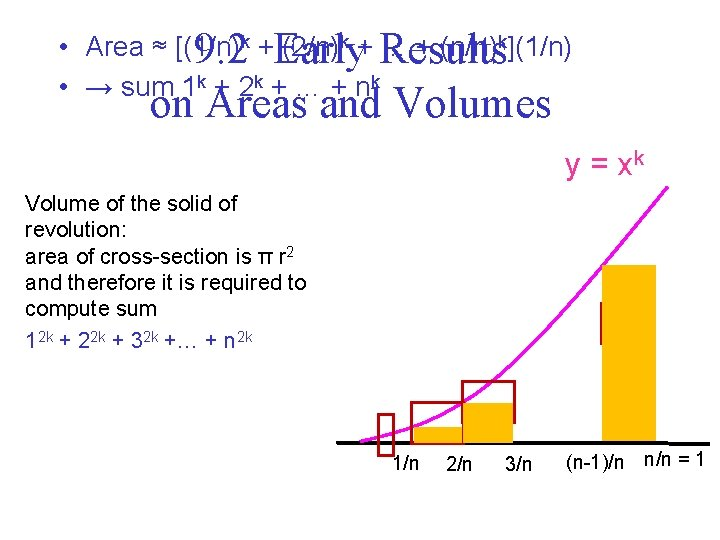k + (2/n)k + … + (n/n)k](1/n) • Area ≈ [(1/n) 9. 2 Early Results • → sum 1 k + 2 k + … + nk on Areas and Volumes y = xk Volume of the solid of revolution: area of cross-section is π r 2 and therefore it is required to compute sum 12 k + 22 k + 32 k +… + n 2 k 1/n 2/n 3/n (n-1)/n n/n = 1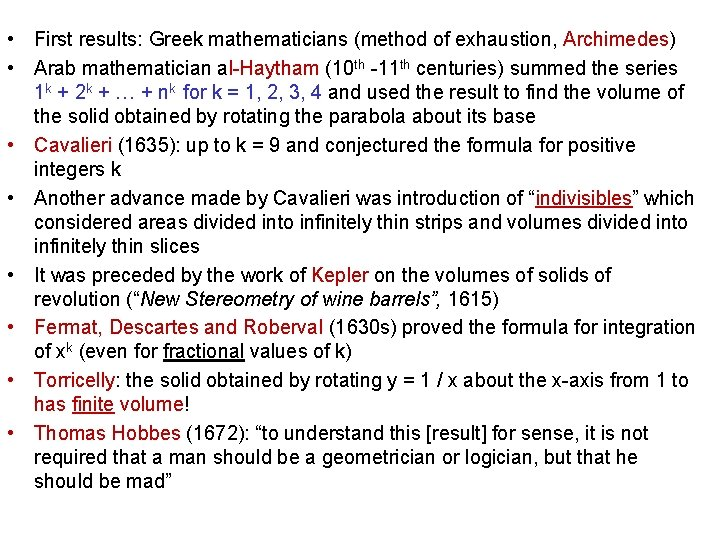• First results: Greek mathematicians (method of exhaustion, Archimedes) • Arab mathematician al-Haytham (10 th -11 th centuries) summed the series 1 k + 2 k + … + nk for k = 1, 2, 3, 4 and used the result to find the volume of the solid obtained by rotating the parabola about its base • Cavalieri (1635): up to k = 9 and conjectured the formula for positive integers k • Another advance made by Cavalieri was introduction of “indivisibles” which considered areas divided into infinitely thin strips and volumes divided into infinitely thin slices • It was preceded by the work of Kepler on the volumes of solids of revolution (“New Stereometry of wine barrels”, 1615) • Fermat, Descartes and Roberval (1630 s) proved the formula for integration of xk (even for fractional values of k) • Torricelly: the solid obtained by rotating y = 1 / x about the x-axis from 1 to has finite volume! • Thomas Hobbes (1672): “to understand this [result] for sense, it is not required that a man should be a geometrician or logician, but that he should be mad”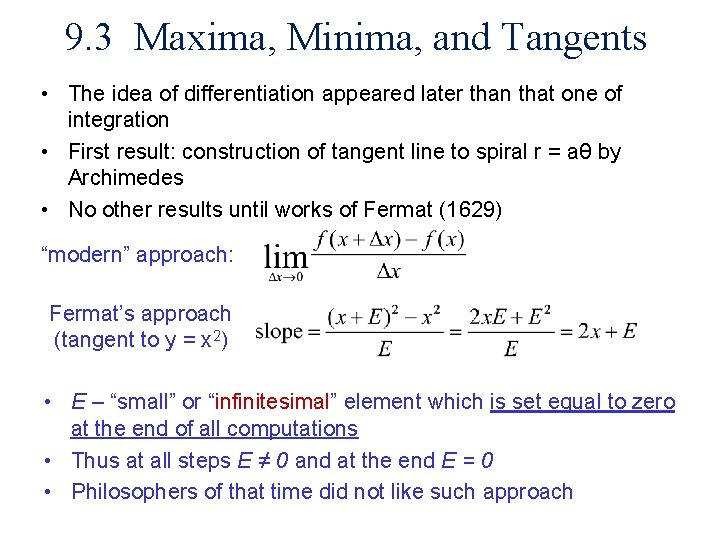9. 3 Maxima, Minima, and Tangents • The idea of differentiation appeared later than that one of integration • First result: construction of tangent line to spiral r = aθ by Archimedes • No other results until works of Fermat (1629) “modern” approach: Fermat’s approach (tangent to y = x 2) • E – “small” or “infinitesimal” element which is set equal to zero at the end of all computations • Thus at all steps E ≠ 0 and at the end E = 0 • Philosophers of that time did not like such approach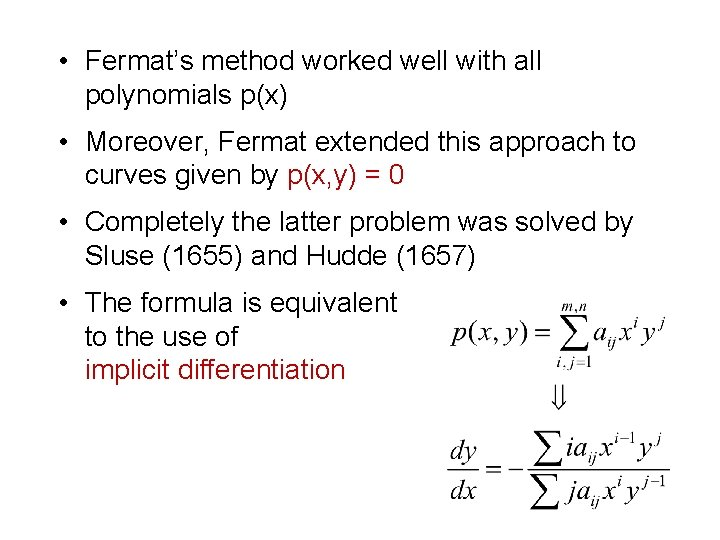• Fermat’s method worked well with all polynomials p(x) • Moreover, Fermat extended this approach to curves given by p(x, y) = 0 • Completely the latter problem was solved by Sluse (1655) and Hudde (1657) • The formula is equivalent to the use of implicit differentiation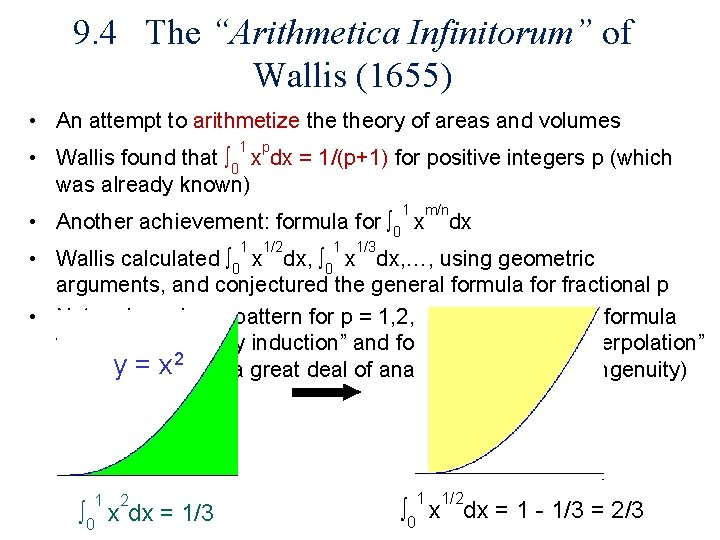9. 4 The “Arithmetica Infinitorum” of Wallis (1655) • An attempt to arithmetize theory of areas and volumes 1 p • Wallis found that ∫ 0 x dx = 1/(p+1) for positive integers p (which was already known) 1 m/n • Another achievement: formula for ∫ 0 x 1 1/2 1 dx 1/3 • Wallis calculated ∫ 0 x dx, …, using geometric arguments, and conjectured the general formula for fractional p • 1 Note: observing a pattern for p = 1, 2, 3, Wallis claimed a formula for all positive p “by induction” and for fractional p “by interpolation” y rigour = x 2 but a great deal of analogy, intuition and ingenuity) (lack of 1 2 ∫ 0 x dx = 1/3 1 1 1/2 ∫ 0 x dx = 1 - 1/3 = 2/3• Wallis’ formula: • Expansion of π as infinite product was known to Viète (before Wallis’ discovery): • Nevertheless Wallis’ formula relates π to the integers through a sequence of rational operations • Moreover, basing on the formula for π Wallis’ found a sequence of fractions he called “hypergeometric”, which as it had been found later occur as coefficients in series expansions of many functions (which led to the class of hypergeometric functions)Other formulas for π related to Wallis’ formula Continued fraction (Brouncker): Series expansion discovered by 15 th century Indian Euler mathematicians and rediscovered by Newton, Gregory and Leibniz: sub. x = 19. 5 Newton’s Calculus of Series • Isaac Newton – – Most important discoveries in 1665/6 Before he studied the works of Descartes, Viète and Wallis Contributions to differential calculus (e. g. the chain rule) Most significant contributions are related to theory of infinite series – Newton used term-by-term integration and differentiation to find power series representation of many of classical functions, such as tan-1 x or log (x+1) – Moreover, Newton developed a method of inverting infinite power series to find inverses of functions (e. g ex from log (x+1)) • Unfortunately, Newton’s works were rejected for publication by Royal Society and Cambridge University Press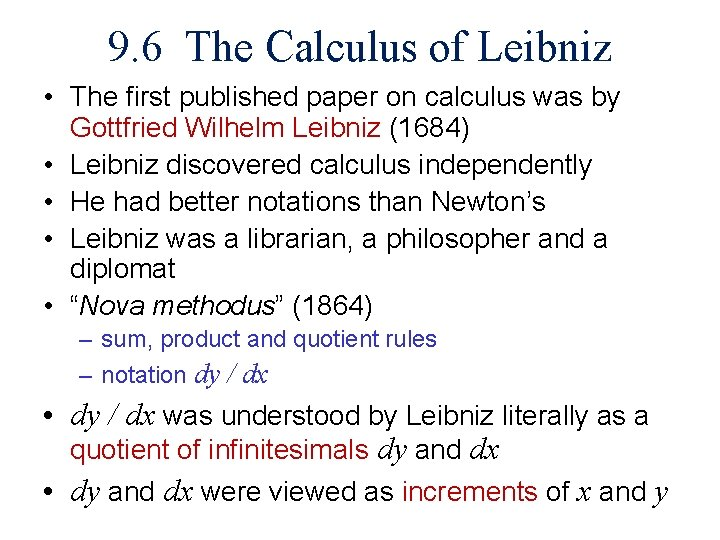9. 6 The Calculus of Leibniz • The first published paper on calculus was by Gottfried Wilhelm Leibniz (1684) • Leibniz discovered calculus independently • He had better notations than Newton’s • Leibniz was a librarian, a philosopher and a diplomat • “Nova methodus” (1864) – sum, product and quotient rules – notation dy / dx • dy / dx was understood by Leibniz literally as a quotient of infinitesimals dy and dx • dy and dx were viewed as increments of x and y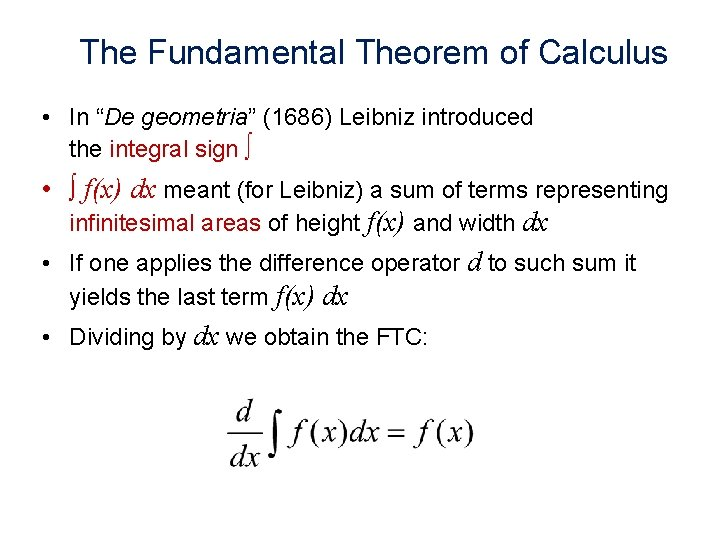The Fundamental Theorem of Calculus • In “De geometria” (1686) Leibniz introduced the integral sign ∫ • ∫ f(x) dx meant (for Leibniz) a sum of terms representing infinitesimal areas of height f(x) and width dx • If one applies the difference operator d to such sum it yields the last term f(x) dx • Dividing by dx we obtain the FTC: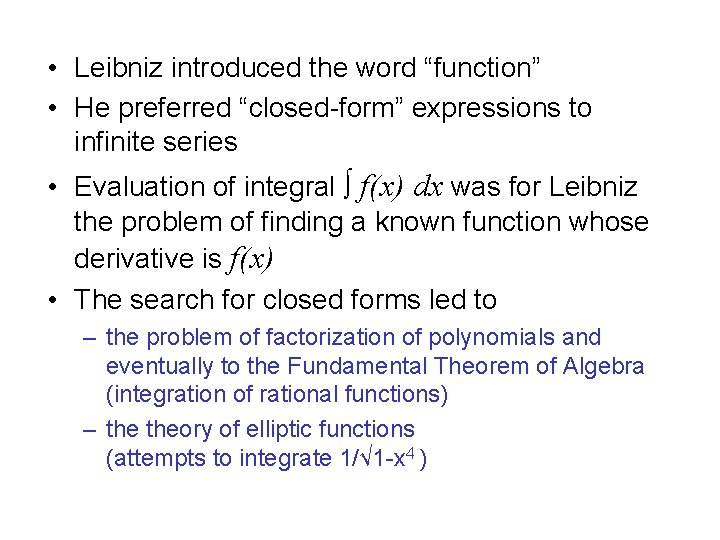• Leibniz introduced the word “function” • He preferred “closed-form” expressions to infinite series • Evaluation of integral ∫ f(x) dx was for Leibniz the problem of finding a known function whose derivative is f(x) • The search for closed forms led to – the problem of factorization of polynomials and eventually to the Fundamental Theorem of Algebra (integration of rational functions) – theory of elliptic functions (attempts to integrate 1/√ 1 -x 4 )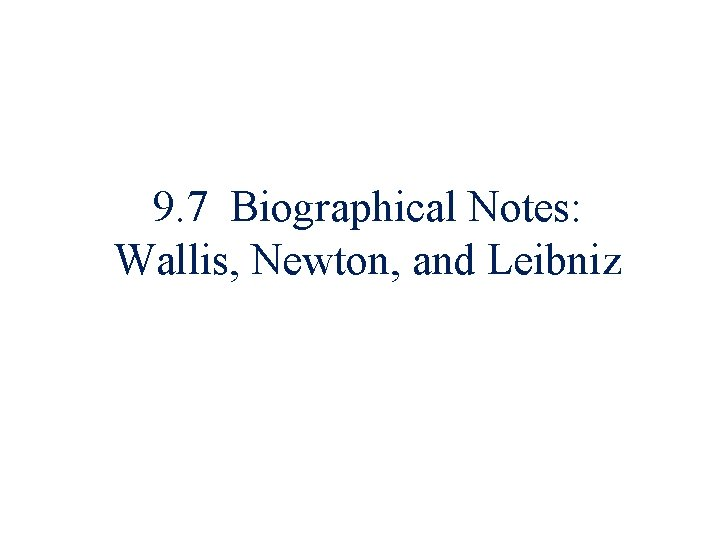9. 7 Biographical Notes: Wallis, Newton, and LeibnizJohn Wallis Born: 23 Nov 1616 (Ashford, Kent, England) Died: 28 Oct 1703 (Oxford, England)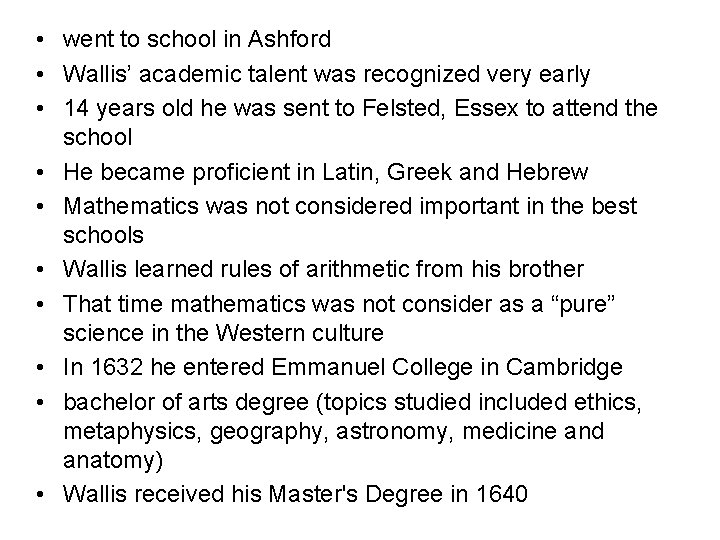• went to school in Ashford • Wallis’ academic talent was recognized very early • 14 years old he was sent to Felsted, Essex to attend the school • He became proficient in Latin, Greek and Hebrew • Mathematics was not considered important in the best schools • Wallis learned rules of arithmetic from his brother • That time mathematics was not consider as a “pure” science in the Western culture • In 1632 he entered Emmanuel College in Cambridge • bachelor of arts degree (topics studied included ethics, metaphysics, geography, astronomy, medicine and anatomy) • Wallis received his Master's Degree in 1640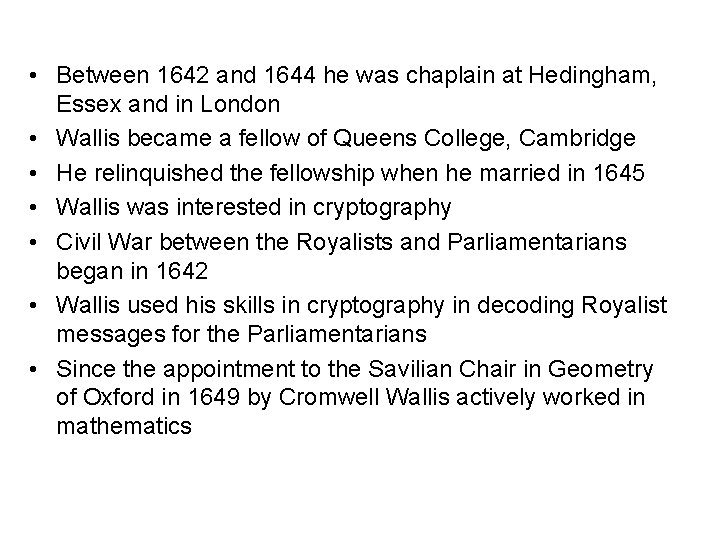• Between 1642 and 1644 he was chaplain at Hedingham, Essex and in London • Wallis became a fellow of Queens College, Cambridge • He relinquished the fellowship when he married in 1645 • Wallis was interested in cryptography • Civil War between the Royalists and Parliamentarians began in 1642 • Wallis used his skills in cryptography in decoding Royalist messages for the Parliamentarians • Since the appointment to the Savilian Chair in Geometry of Oxford in 1649 by Cromwell Wallis actively worked in mathematics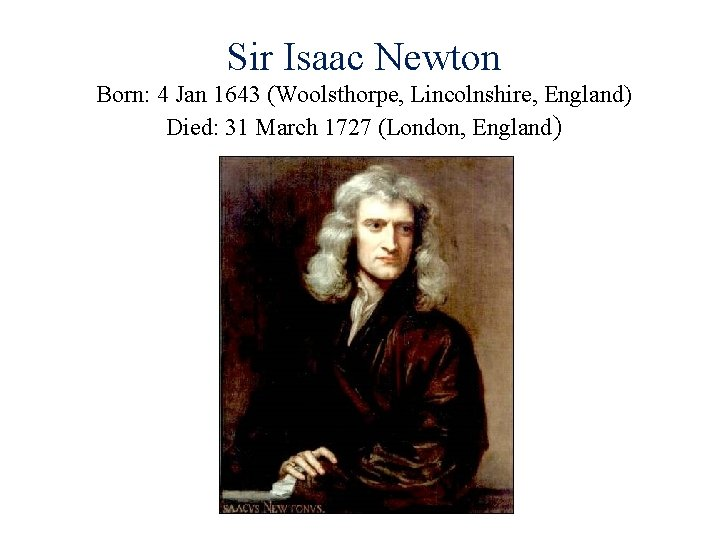Sir Isaac Newton Born: 4 Jan 1643 (Woolsthorpe, Lincolnshire, England) Died: 31 March 1727 (London, England)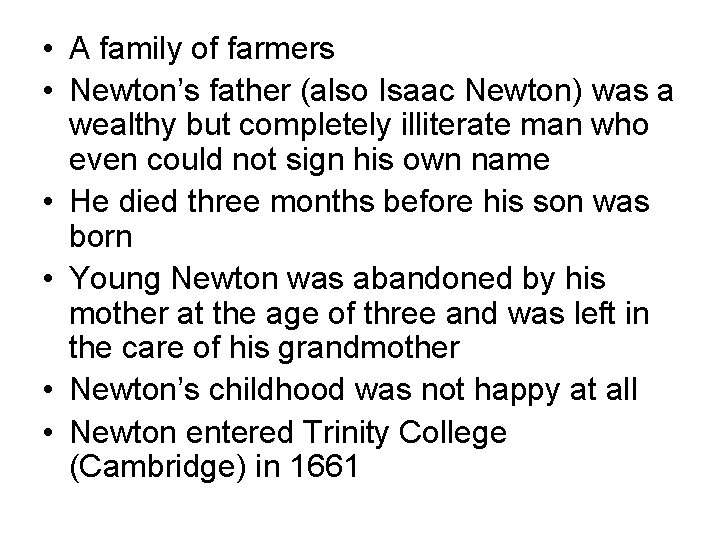• A family of farmers • Newton’s father (also Isaac Newton) was a wealthy but completely illiterate man who even could not sign his own name • He died three months before his son was born • Young Newton was abandoned by his mother at the age of three and was left in the care of his grandmother • Newton’s childhood was not happy at all • Newton entered Trinity College (Cambridge) in 1661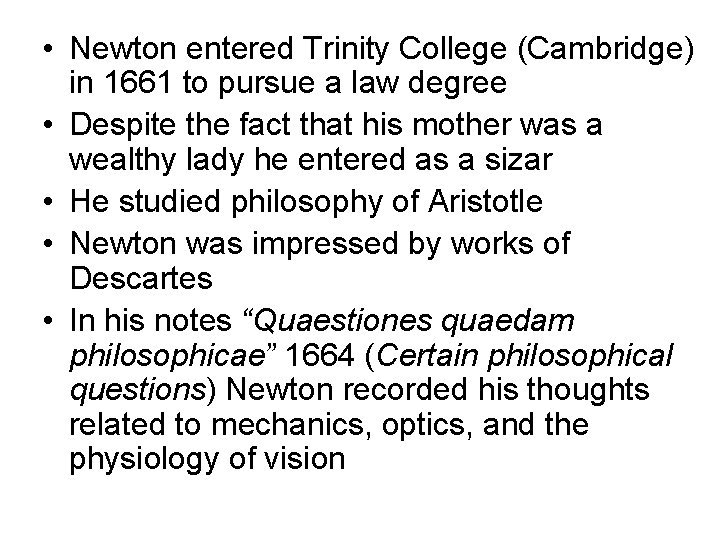• Newton entered Trinity College (Cambridge) in 1661 to pursue a law degree • Despite the fact that his mother was a wealthy lady he entered as a sizar • He studied philosophy of Aristotle • Newton was impressed by works of Descartes • In his notes “Quaestiones quaedam philosophicae” 1664 (Certain philosophical questions) Newton recorded his thoughts related to mechanics, optics, and the physiology of vision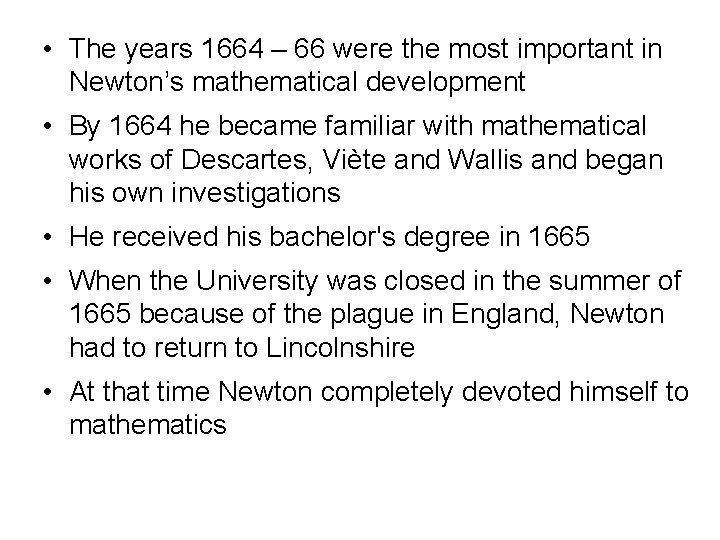• The years 1664 – 66 were the most important in Newton’s mathematical development • By 1664 he became familiar with mathematical works of Descartes, Viète and Wallis and began his own investigations • He received his bachelor's degree in 1665 • When the University was closed in the summer of 1665 because of the plague in England, Newton had to return to Lincolnshire • At that time Newton completely devoted himself to mathematics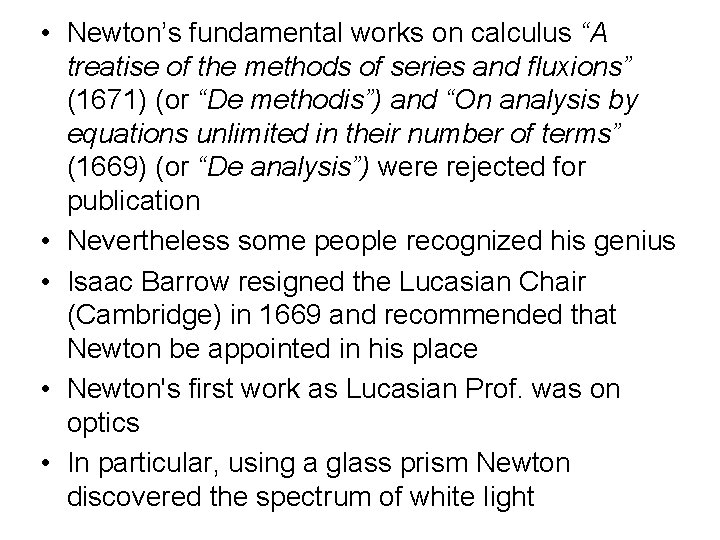• Newton’s fundamental works on calculus “A treatise of the methods of series and fluxions” (1671) (or “De methodis”) and “On analysis by equations unlimited in their number of terms” (1669) (or “De analysis”) were rejected for publication • Nevertheless some people recognized his genius • Isaac Barrow resigned the Lucasian Chair (Cambridge) in 1669 and recommended that Newton be appointed in his place • Newton's first work as Lucasian Prof. was on optics • In particular, using a glass prism Newton discovered the spectrum of white light• 1665: Newton discovered inverse square law of gravitation • 1687: “Philosophiae naturalis principia mathematica” (Mathematical principles of natural philosophy) • In this work, Newton developed mathematical foundation of theory of gravitation • This book was published by Royal Society (with the strong support from Edmund Halley) • In 1693 Newton had a nervous breakdown • In 1696 he left Cambridge and accepted a government position in London where he became master of the Mint in 1699 • In 1703 he was elected president of the Royal Society and was re-elected each year until his death • Newton was knighted in 1705 by Queen AnneGottfried Wilhelm von Leibniz Born: 1 July 1646 (Leipzig, Saxony (now Germany) Died: 14 Nov 1716 (Hannover, Hanover (now Germany)• An academic family • From the age of six Leibniz was given free access to his father’s library • • At the age of seven he entered school in Leipzig In school he studied Latin Leibniz had taught himself Latin and Greek by the age of 12 He also studied Aristotle's logic at school In 1661 Leibniz entered the University of Leipzig He studied philosophy and mathematics In 1663 he received a bachelor of law degree for a thesis “De Principio Individui” (“On the Principle of the Individual”) • The beginning of the concept of “monad” • He continued work towards doctorate • Leibniz received a doctorate degree from University of Altdorf (1666)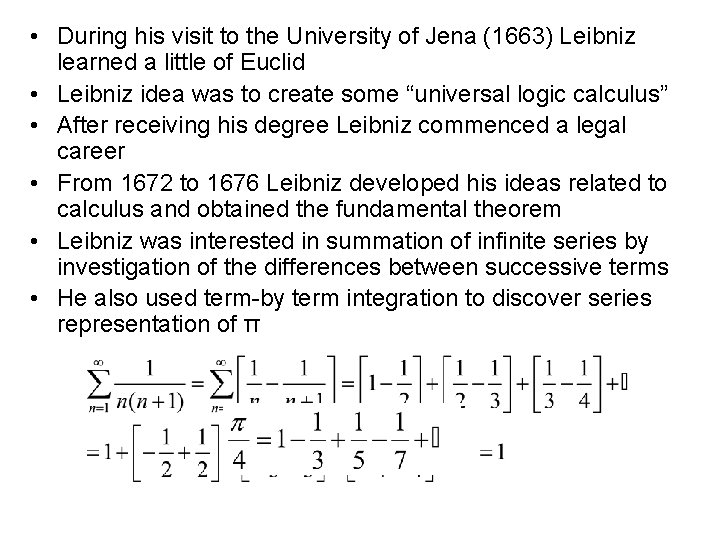• During his visit to the University of Jena (1663) Leibniz learned a little of Euclid • Leibniz idea was to create some “universal logic calculus” • After receiving his degree Leibniz commenced a legal career • From 1672 to 1676 Leibniz developed his ideas related to calculus and obtained the fundamental theorem • Leibniz was interested in summation of infinite series by investigation of the differences between successive terms • He also used term-by term integration to discover series representation of π